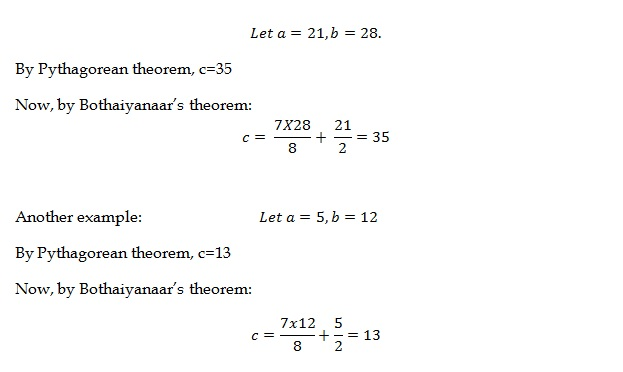# An Alternative to the Pythagorian Theorem by a Keralite Mathematics Teacher

I rose to read Mathrubhumi daily today, only to amuse myself for a Keralite Mathematics teacher – Mr. Rahumathulla – has devised an alternative to the well-known Pythagorian Theorem. The original theorem is supposed to be so minimalistic and unimprovable that even the fifth graders in our schools are given lectures on the same.

But, removing the concept of square root, Mr. Rahmathulla has devised a new equation, which might seem big in size, but simpler in calculations (especially when bigger numbers are involved and the complexity of the operations are so high because of the square root function). Hats off to Mr. Rahumathulla. As a Math enthusiast, I couldn’t help posting it on my blog.

Link to the news (text in Malayalam): http://digitalpaper.mathrubhumi.com/c/2570066

The proof of this finding as published by The Association of Mathematics Teachers of India, Chennai is given below (thanks to Mr. Rahumathulla for sending me this).

(Click on the images to view in higher resolution)

Update: Bothaiyanaar’s Alternative to Pythagorean Theorem

Original post: http://taaism.com/bothaiyanaar-alternative-to-pythagorean-theorem

It was quite accidentally, when reading about Pythagorean theorem, that I stumbled upon this amazingly simpler theorem which stands as an alternative to Pythagorean theorem. This was actually written by a Tamil poet – Bothaiyanaar – and is useful while calculating the hypotenuse of a right angled triangle. Note that this theorem works well when the numbers involved are whole numbers. When the hypotenuse is not a whole number, the theorem seems to be giving not accurate, but approximate values. The following is a stanza from Bothaiyanaar’s poem (in Tamil script 1):

“ஓடும் நீளம் தனை ஒரேஎட்டுக்
கூறு ஆக்கி கூறிலே ஒன்றைத்
தள்ளி குன்றத்தில் பாதியாய்ச் சேர்த்தால்
வருவது கர்ணம் தானே”

This 2 means: Divide the distance you’ve run (longer side) into 8 equal parts and discard one part from it. Then if you add the half the height to that, you’ll get Hypotenuse.

The advantage of the theorem is that we need not perform the square root function, which might be a hazard for the little school students. That way, this works fine for them. Let’s see how it works:

Consider two smaller sides, say a and b, of a right-angled triangle. Let $a \leq b$. Then,

[latex size=”4″] hyp = \frac{7b}{8}+\frac{a}{2}[/latex]

Let’s discuss two examples now:Further, this trick seems to be applicable for the normal pythagorean triplets of the form (3x, 4x, 5x) and (5x, 12x, 13x). It doesn’t seem to be okay for (7x, 24x, 25x), where it gives not an accurate answer.

Thanks to Manish Bansal for a quick proof as to why this works, that’s given below:

Let’s assume that this theorem is true for all whole numbers, as far as a right angled triangle is concerned. On comparing the hypotenuse of this theorem and traditional Pythagorean theorem:

[latex size=”2″]\Rightarrow a^{2}+b^{2} = \left ( \frac{7b}{8} + \frac{a}{2} \right )^{2}[/latex]

[latex size=”2″]\Rightarrow a^{2}+b^{2} = \frac{49b^{2}}{64}+\frac{7ab}{8}+\frac{a^{2}}{4}[/latex]

Multiplying both sides by 64,

[latex size=”2″]\Rightarrow 64a^{2} + 64b^{2} = 49b^{2}+56ab+16a^{2}[/latex]

[latex size=”2″]\Rightarrow 48 a^{2}+15b^{2}=56ab[/latex]

Dividing both sides by $latex ab$,

[latex size=”2″]\Rightarrow 48\frac{a}{b}+15\frac{b}{a}=56[/latex]

Let z = a/b.

[latex size=”2″]\Rightarrow 48z+\frac{15}{z}=56[/latex]

Multiplying by z,

[latex size=”2″]\Rightarrow 48z^{2}-56z+15=0[/latex]

Applying the basic equation to solve the above quadratic equation,

[latex size=”2″]\Rightarrow z=\frac{56\pm \sqrt{56\times 56-4\times 48\times 15}}{2\times 48}[/latex]

[latex size=”2″]\Rightarrow z=\frac{5}{12}, \frac{3}{4}[/latex]

This shows that the ratio a/b, is either 3/4 or 5/12. Only then, it will give proper results for the hypotenuse. If the ratio is 7/24, this theorem will not give accurate result as that’s not a root of the above quadratic equation. The theorem as such might not look promising, but it is nice to have a thought on the simplicity of this theorem, though having limited application.

Thanks,

Arunanand T A

Notes:

1. http://ramsundarvijayraj.blogspot.in/2011/12/bothaiyanaar-pythagoras-theorem.html
2. www.durofy.com/alternate-pythagoras-theorem